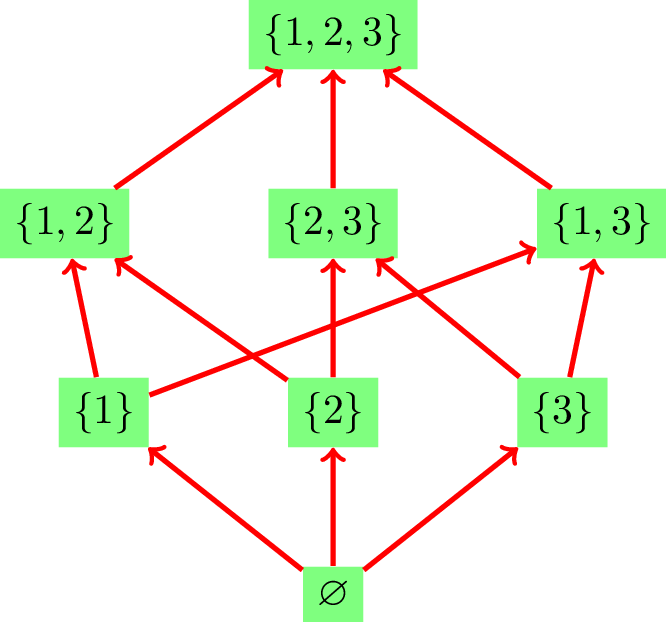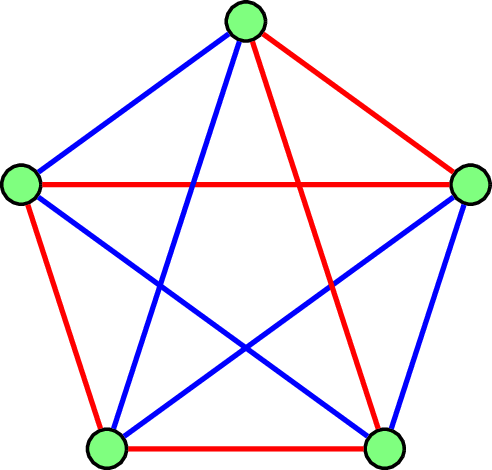$$\require{physics} \def\*#1{\mathbf{#1}} \def\+#1{\mathcal{#1}} \def\-#1{\mathrm{#1}}\def\^#1{\mathbb{#1}}\def\!#1{\mathtt{#1}} \def\@#1{\mathscr{#1}} \newcommand{\norm}{\left\Vert#1\right\Vert} \newcommand{\set}{\left\{\,#1\,\right\}} \newcommand{\tp}{\left(#1\right)} \newcommand{\eps}{\varepsilon} \newcommand{\inner}{\langle #1,#2\rangle} \renewcommand{\mid}{\;\middle\vert\;} \newcommand{\cmid}{\,:\,} \newcommand{\numP}{\#\mathbf{P}} \renewcommand{\P}{\mathbf{P}} \newcommand{\defeq}{\triangleq} \newcommand{\ol}{\overline} \newcommand{\Pr}[]{\mathbf{Pr}_{#1}\left[#2\right]} \newcommand{\E}[]{\mathbf{E}_{#1}\left[#2\right]} \newcommand{\Var}[]{\mathbf{Var}_{#1}\left[#2\right]} \renewcommand{\emptyset}{\varnothing} \renewcommand{\d}{\dd} \newcommand{\mle}{\preceq} \newcommand{\mge}{\succeq} \newcommand{\DKL}{D_{\!{KL}}\tp{#1\,\|\,#2}} \newcommand{\DTV}{D_{\!{TV}}\tp{#1\,\|\,#2}} \newcommand{\argmin}{\mathop{\arg\min}} \newcommand{\argmax}{\mathop{\arg\max}} \newcommand{\eps}{\varepsilon} \def\multiset#1#2{\left(\kern-.2em\left(\genfrac{}{}{0pt}{}{#1}{#2}\right)\kern-.2em\right)} % define stirling number \newcommand{\genstirlingI}{% \genfrac{[}{]}{0pt}{#1}{#2}{#3}% } \newcommand{\genstirlingII}{% \genfrac{\{}{\}}{0pt}{#1}{#2}{#3}% } \newcommand{\stirlingI}{\genstirlingI{}{#1}{#2}} \newcommand{\dstirlingI}{\genstirlingI{0}{#1}{#2}} \newcommand{\tstirlingI}{\genstirlingI{1}{#1}{#2}} \newcommand{\stirlingII}{\genstirlingII{}{#1}{#2}} \newcommand{\dstirlingII}{\genstirlingII{0}{#1}{#2}} \newcommand{\tstirlingII}{\genstirlingII{1}{#1}{#2}} % end of defining stirling number$$

# 第一讲：课程简介

Published

February 18, 2023

• 在组合数学以及计算机科学中反复出现的结构（structure）、现象（phenomenon）以及问题（problem）；
• 能够用来尝试解决问题的基本方法与技术。

# 结构与问题

## 计数与枚举

• 具有 $$n$$ 个（有编号的）顶点的不同的树的个数是 $$n^{n-2}$$ （Cayley公式）。
• 斐波那契（Fibonacci）数列是满足 $$f_0=0; f_1=1; f_n=f_{n-1}+f_{n-2}, n\ge 2$$ 的一组数。可以得到它的通项是 $$f_n = \frac{1}{\sqrt{5}}\tp{\phi_1^n-\phi_2^n}$$，其中 $$\phi_1 = \frac{1+\sqrt{5}}{2}$$$$\phi_2 = \frac{1-\sqrt{5}}{2}$$
• 计算一个二分图 $$G=(V_1\cup V_2, E)$$ 上的完美匹配数。假设 $$\abs{V_1}=\abs{V_2}=n$$。如果我们用 $$I\in\set{0,1}^{n\times n}$$ 表示其相邻矩阵（incidence matrix），即 $$I(i,j)=1$$ 当且仅当二分图左边的第 $$i$$ 个顶点和右边第 $$j$$ 个顶点有一条边相邻。那么 $$G$$ 中完美匹配的个数为 $\sum_{\sigma \in S_n}\prod_{i=1}^n I(i,\sigma(i)).$

$$\-{FP}$$ 即我们通常说的多项式时间可以计算的函数，它与 $$\-{P}$$ 的区别是后者通常指的是判定性问题。而 $$\#\-P$$ 指的是那些可以用多项式时间的非确定性图灵机的接受路径个数来表示的计算函数。

## 偏序结构

• 自反性（Reflexivity）：$$\forall x\in P\colon x\le x$$
• 反对称性（Antisymmetric）：$$\forall x,y\in P\colon x\le y \land y\le x\implies x=y$$
• 传递性（Transitivity）：$$\forall x,y,z\in P\colon x\le y \land y\le z\implies x\le z$$Figure 1: $$(2^{},\subseteq)$$的 Hasse 图

Definition 1

Definition 2

Figure 1 中，$$T=\set{\set{1},\set{2},\set{3}}$$ 就是一个反链。一个偏序集中最长反链的长度被称之为这个偏序集的宽度（width）Figure 1 中偏序集的宽度是$$3$$

Definition 3 假设 $$C_1,C_2,\dots,C_m$$ 都是偏序集 $$(P,\le)$$ 上的链。我们说它们构成一个链覆盖（chain cover），如果满足：

• $$\bigcup_{i\in [m]} C_i = P$$，即这些链覆盖了 $$P$$ 中所有元素；
• $$\forall i,j\in [m]\colon i\ne j\implies C_i\cap C_j=\emptyset$$，即这些链是不相交的。

Definition 4 假设 $$T_1,T_2,\dots,T_p$$ 都是偏序集 $$(P,\le)$$ 上的反链。我们说它们构成一个反链覆盖（antichain cover），如果满足：

• $$\bigcup_{i\in [p]} T_i = P$$，即这些反链覆盖了 $$P$$ 中所有元素；
• $$\forall i,j\in [p]\colon i\ne j\implies T_i\cap T_j=\emptyset$$，即这些反链是不相交的。

Theorem 1 (Dilworth)

• 任何一个偏序集的最小链覆盖的大小等于它的宽度。
• 任何一个偏序集中的最小反链覆盖的大小等于它的高度。

Dilworth 定理是一个典型的所谓 min-max 定理，或者被称为对偶定理。这种类型的定理在组合数学中是很多的。我们也将在后续的课程中证明其和König’s 定理以及Hall’s Marriage 定理是等价的。并且，它们在某种意义下都可以看成是线性规划的强对偶定理的推论。

## 极值组合学（extremal combinatorics）(a) A coloring of $$K_5$$

Proposition 1

Theorem 2 (Erdős-Szekeres)

Proof.

$$N=R(r-1,s)+R(r,s-1)$$，我们只需要证明，对于 $$K_N$$的任何一个红蓝染色中，要么存在红色 $$K_r$$，要么存在蓝色 $$K_s$$。现在固定一个染色，考察点 $$1$$ 相邻的所有 $$N-1$$ 条边 $$\set{1,2},\set{1,3},\dots,\set{1,N}$$ 的颜色。根据鸽笼原理，要么存在至少 $$R(r-1,s)$$ 条红边，要么存在 $$R(r,s-1)$$ 条蓝边。

Theorem 3 (Erdős)

# 方法与技术

Proof.

## 代数方法（algebraic methods）

Definition 5

• 使用矩阵 $$D_G\defeq\!{diag}(\!{deg}(1),\!{deg}(2),\dots,\!{deg}(n))$$ 表示以图的度数为对角线元素的对角矩阵。我们称 $$D_G$$$$G$$度数矩阵
• 矩阵 $$L_G\defeq D_G-A_G$$ 被称为图 $$G$$拉普拉斯（Laplacian）矩阵，有时候简称为 $$G$$ 的 Laplacian。

Theorem 4

Proof.